# Three-Dimensional plotting

Python allows to build 3D charts thanks to the `mplot3d` toolkit of the `matplotlib` library. However, please note that 3d charts are most often a bad practice. This section focuses on 3d scatter plots and surface plots that are some interesting use cases.

## ⏱ Quick start

``````# libraries
from mpl_toolkits.mplot3d import Axes3D
import matplotlib.pyplot as plt
import numpy as np
import pandas as pd

# Dataset
df=pd.DataFrame({'X': range(1,101), 'Y': np.random.randn(100)*15+range(1,101), 'Z': (np.random.randn(100)*15+range(1,101))*2 })

# plot
fig = plt.figure()
ax.scatter(df['X'], df['Y'], df['Z'], c='skyblue', s=60)
ax.view_init(30, 185)
plt.show()
``````

##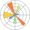Three-dimensional scatterplots with `Matplotlib`

As described in the quick start section above, a three dimensional can be built with python thanks to the`mplot3d` toolkit of `matplotlib`. The example below will guide you through its usage to get this figure: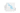Basic 3d scatterplot with Python & Matplotlib.

This technique is useful to visualize the result of a PCA (Principal Component Analysis). The following example explains how to run a PCA with python and check its result with a 3d scatterplot: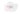PCA result shown as a 3D scatterplot with python

##Surface plot with `Matplotlib`

A surface plot considers the X and Y coordinates as latitude and longitude, and Z as the altitude. It represents the dataset as a surface by interpolating positions between data points.

This kind of chart can also be done thanks to the `mplot3d` toolkit of `matplotlib`. The posts linked below explain how to use and customize the `trisurf()` function that is used for surface plots.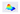Basic 3d scatterplot with Python & Matplotlib.

##Three dimensional plot and animation

You can build an animation from a 3d chart by changing the camera position at each iteration of a loop. The example below explains how to do it for a surface plot but visit the animation section for more.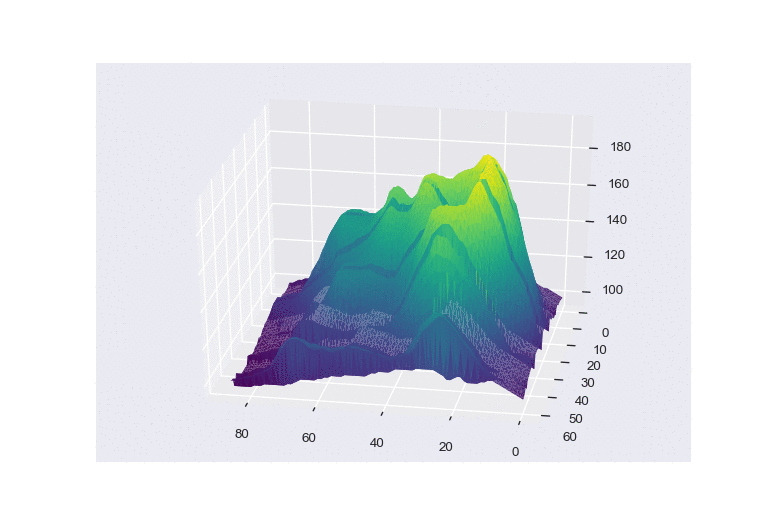## Contact

👋 This document is a work by Yan Holtz. You can contribute on github, send me a feedback on twitter or subscribe to the newsletter to know when new examples are published! 🔥